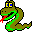#Boost C++ Libraries

...one of the most highly regarded and expertly designed C++ library projects in the world.#topological_sort

```template <typename VertexListGraph, typename OutputIterator,
typename P, typename T, typename R>
void topological_sort(VertexListGraph& g, OutputIterator result,
const bgl_named_params<P, T, R>& params = all defaults)
```

### Where Defined:

boost/graph/topological_sort.hpp

### Parameters

IN: VertexListGraph& g
A directed acylic graph (DAG). The graph type must be a model of Vertex List Graph and Incidence Graph. If the graph is not a DAG then a not_a_dag exception will be thrown and the user should discard the contents of result range.
Python: The parameter is named graph.
OUT: OutputIterator result
The vertex descriptors of the graph will be output to the result output iterator in reverse topological order. The iterator type must model Output Iterator.
Python: This parameter is not used in Python. Instead, a Python list containing the vertices in topological order is returned.

### Named Parameters

UTIL/OUT: color_map(ColorMap color)
This is used by the algorithm to keep track of its progress through the graph. The type ColorMap must be a model of Read/Write Property Map and its key type must be the graph's vertex descriptor type and the value type of the color map must model ColorValue.
Default: an iterator_property_map created from a std::vector of default_color_type of size num_vertices(g) and using the i_map for the index map.
Python: The color map must be a vertex_color_map for the graph.
IN: vertex_index_map(VertexIndexMap i_map)
This maps each vertex to an integer in the range [0, num_vertices(g)). This parameter is only necessary when the default color property map is used. The type VertexIndexMap must be a model of Readable Property Map. The value type of the map must be an integer type. The vertex descriptor type of the graph needs to be usable as the key type of the map.
Default: get(vertex_index, g) Note: if you use this default, make sure your graph has an internal vertex_index property. For example, adjacency_list with VertexList=listS does not have an internal vertex_index property.
Python: Unsupported parameter.

### Complexity

The time complexity is O(V + E).

### Example

Calculate a topological ordering of the vertices.

```  typedef adjacency_list< vecS, vecS, directedS, color_property<> > Graph;
typedef boost::graph_traits<Graph>::vertex_descriptor Vertex;
Pair edges = { Pair(0,1), Pair(2,4),
Pair(2,5),
Pair(0,3), Pair(1,4),
Pair(4,3) };
Graph G(6, edges, edges + 6);

typedef std::vector< Vertex > container;
container c;
topological_sort(G, std::back_inserter(c));

cout << "A topological ordering: ";
for ( container::reverse_iterator ii=c.rbegin(); ii!=c.rend(); ++ii)
cout << index(*ii) << " ";
cout << endl;
```
The output is:
```  A topological ordering: 2 5 0 1 4 3
```

 Copyright © 2000-2001 Jeremy Siek, Indiana University (jsiek@osl.iu.edu)